# HC Verma Solutions Class 11 Chapter 18 Geometrical Optics

HC Verma Solutions Class 11 Chapter 18 Geometrical Optics provides a thorough and contemporary approach to understand questions related to the mirror equation, finding the focal length and magnification, etc. The solutions contain accurate answers that describe all the major questions which are discussed in class 11 HC Verma book and questions that are often seen in competitive examinations like IIT JEE. Students will learn the right method to solve problems from the chapter like;

• Questions on finding the focal length and the image distance formed from the mirror along with the image’s nature.
• Problems on celestial objects with their diameter given and find out where the image will be formed.
• Questions on the real and apparent depth as well as concepts of light travelling in a glass slab and the shifting due to the glass slab.
• Questions related to finding the shift when the refractive index of multiple slabs is given and position of the image.
• Some of the other questions are based on finding the critical angle of glass when total internal reflection is given as well as finding the angle of deviation when the angle of incidence and refraction are given.

The HC Verma solutions is a great tool that will help students to not only find the right answers but also understand the concept more clearly. Study more effectively and stay ahead in the competition by referring to the solutions provided here.

Students will also get to know more about topics such as;

1. Reflection at Smooth Surfaces
2. Spherical Mirrors
3. Relation Between u, v and R for Spherical Mirrors
4. Extended Objects and Magnification
5. Refraction at Plane Surfaces
6. Critical Angle
7. Optical Fibre
8. Prism
9. Refraction at Spherical Surfaces
10. Extended Objects: Lateral Magnification
11. Refraction through Thin Lenses
12. Lens Maker’s Formula and Lens Formula
13. Extended Objects: Lateral Magnification
14. Power of a Lens Thin Lenses in Contact
15. Two Thin Lenses Separated By a Distance
16. Defects of Images

## Class 11 Important Questions In Chapter 18

1. Do you think that a camera can be used to photograph a virtual image?

2. What happens to the focal length of a spherical mirror or a thin lens when they are dipped in water? What changes do you notice?

3. A light source is kept at a distance of 2 f from a converging lens having a focal length 1. Where will the intensity of light on the other side of the lens be maximum at?

(a) 2 f (b) f (c) between f and 2 f (d) greater than 2 f

4. Taking symmetric double convex lens we cut it in two equal parts with a plane that is perpendicular to the principal axis. The original lens had a power of 4 D. What will the power of a cutlens be?

(a) 5 D (b) 4 D (c) 3 D (d) 2 D.

5. What is the main condition for an image to be erect when it is placed perpendicular to the principal axis of a mirror?

(a) When the object and the image are both real
(b) If the object and the image are both virtual
(c) The object is real but the image is virtual
(d) Or when the object is virtual but the image is real

## HC Verma Solutions Vol 1 Geometrical Optics Chapter 18

SIGN CONVENTION:

(1) The direction of incident ray is taken as positive direction.

(2) All measurements are taken from pole (mirror) or optical centre (lens) as the case may be.

Question 1: A concave mirror having a radius of curvature 40 cm is placed in front of an illuminated point source at a distance of 30 cm from it. Find the location of the image.

Solution:

Here u = -30 cm and R = -40 cm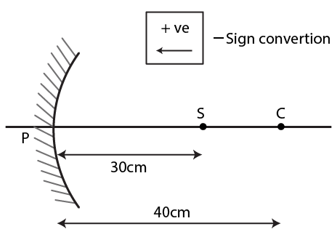From the mirror equation,

1/v + 1/u = 2/R

=> 1/v = 2/-40 – 1/-30 = 1/60

Therefore, the image will be formed at the distance of 60 cm in front of the mirror.

Question 2: A concave mirror forms an image of 20 cm high object on a screen placed 5.0 m away from the mirror. The height of the image is 50 cm. Find the focal length of the mirror and the distance between the mirror and the object.

Solution:

Height of the image = h1= 20cm

Height of the image = h2 = 50cm

The distance of screen from the mirror = v = 5.0 m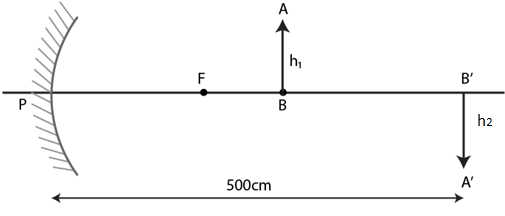Since, -v/u = h2/h1

=> u = [-500×2]/5

= -200 cm = 2 m

From the mirror formula,

1/v + 1/u = 1/f

=> 1/-5 + 1/-2 = 1/f

=> f = -1.44 m -> [focal length]

Question 3: A concave mirror has a focal length of 20 cm. Find the position or positions of an object for which the image-size is double of the object-size.

Solution:

Focal length of the concave mirror = f = -20cm

Magnification of the mirror = M = v/u = 2

=> v = 2u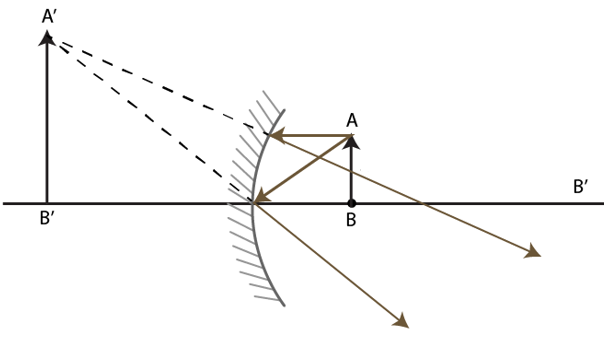Let us consider the image formed will be virtual and beyond the mirror.

Hence, v = -20u

From the mirror formula,

1/v + 1/u = 1/f

=> 1/-2u + 1/u = 1/-20

u = 10 cm

When image formed will be real and inverted. Hence, v = 20u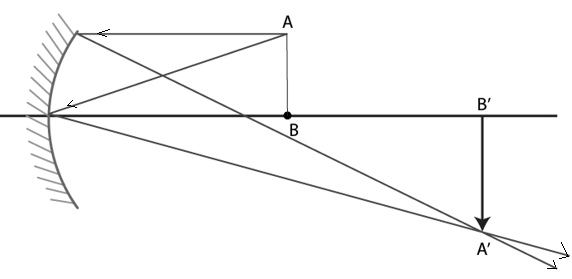=> 1/2u + 1/u = 1/20

u = 30 cm

Therefore, the positions are 10 cm and 30 cm from the concave mirror.

Question 4: A 1 cm object is placed perpendicular to the principal axis of a convex mirror of focal length 7.5 cm. Find its distance from the mirror if the image formed is 0.6 cm in size.

Solution:

Here f = 7.5 cm and M(Magnification) = 0.6 cm = v/u

=> v = 0.6u

From the mirror formula,

1/v + 1/u = 1/f

=> 1/0.6u – 1/u = 1/7.5

u = 5 cm

Question 5: A candle flame 1.6 cm high is imaged in a ball bearing of diameter 0.4 cm. If the ball bearing is 20 cm away from the flame, find the location and the height of the image.

Solution:

The distance of object from the ball =u = 20cm

Diameter of ball bearing= d= 0.4cm

Height of the object = h= 1.6 cm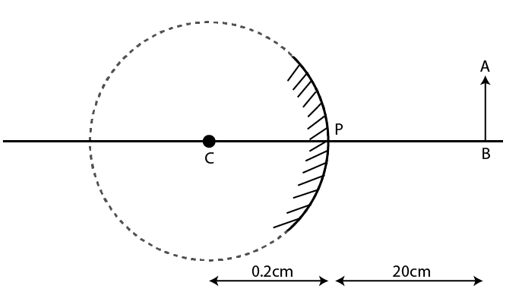Consider distance of the object from the ball = u=-20cm

From the mirror formula,

1/v + 1/-20 = 2/0.2

=> v = 0.1 cm

Now, Magnification= M = -v/u = 0.1/-20 = 1/200

So, height of the image = 16/200 = 0.8 m = 0.8 mm

Question 6: A 3 cm tall object is placed at a distance of 7.5 cm from a convex mirror of focal length 6 cm. Find the location, size and nature of the image.

Solution:

AB = 3 cm, u = -7.5 cm and f = 6 cm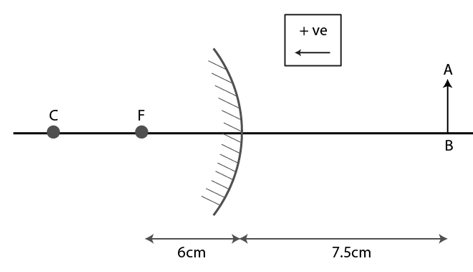From the mirror formula,

1/v + 1/u = 1/f

1/v + 1/-7.5 = 1/6

=> v = 10/3 cm

Now, Magnification= M = -v/u = 10/[7.5×3]

=> A’B’/AB = 10/[7.5×3]

=> A’B’ = 100/72 = 1.33 cm

Image will form at a distance of 10/3 cm. From the pole and image is 1.33 cm.

Question 7: A U-shaped wire is placed before a concave mirror having radius of curvature 20 cm as shown in figure (below). Find the total length of the image.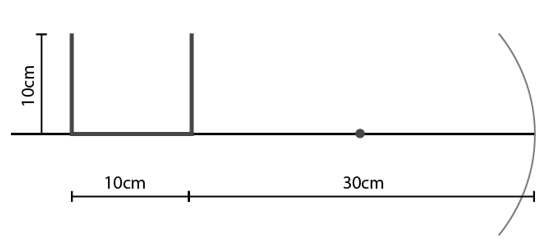Solution:

R = 20 cm, f = R/2 = -10 cm

For part AB, PB = 30+10 =40 cm

Here u = – 40 cm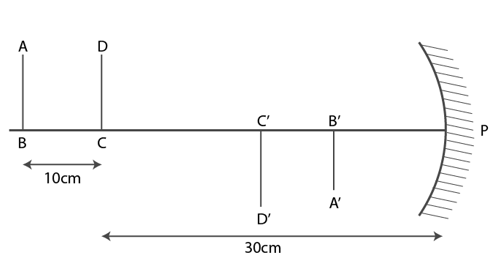From the mirror equation,

1/v + 1/u = 1/f

1/v + 1/-40 = 1/-10

=> v = -13.3 cm

So, PB’ = 13.3 cm

Now, Magnification= M = -v/u = -13.3/-40 = -1/3

=> A’B’= -10/3 cm

For part CD, PC = 30 cm

Here u = – 30 cm

From the mirror equation,

1/v + 1/u = 1/f

1/v + 1/-30 = 1/-10

=> v = -15 cm

So, PC’ = -15 cm

Now, Magnification= M = -v/u = -15/-30 = -1/2

=> C’D’ = 5 cm

Now, B’C’ = PC’- PB’ = 15 – 13.3 = 17 cm

Therefore, total length = A’B’ + B’C’ + C’D’ = 3.3 + 1.7 + 5 = 10 cm

Question 8: A man uses a concave mirror for shaving. He keeps his face at a distance of 25 cm from the mirror and gets an image which is 1.4 times enlarged. Find the focal length of the mirror.

Solution:

Here u = 25 cm

Magnification in the image = 1.4

=> A’B’/AB = -v/u

=> 1.4 = -v/-25

=> v = 35 cm

Using mirror formula,

1/v + 1/u = 1/f

=> 1/f = 1/35 + 1/25 = -2/175

f = -87.5 cm

Which is focal length of the concave mirror.

Question 9: Find the diameter of the image of the moon formed by a spherical concave mirror of focal length 7.6 m. The diameter of the moon is 3450 km and the distance between the earth and the moon is 3.8 x 105 km.

Solution:

Here f = 7.6 m and diameter of the moon is 3450 km

Using mirror formula,

1/v + 1/u = 1/f

using u = – 3.8 x 10^5 km and f = – 7.6 m

=> v = -7.6 cm

Since distance of moon from the earth is very large as compared to focal length it can be taken as infinity.

Now, Magnification, m = -v/u = [distance of the image]/[distance of the object]

=> -(-76)/[- 3.8 x 105] = [distance of the image]/[3450×10^3]

=> Distance of the image = 0.069 m = 6.9 cm

Question 10: A particle goes in a circle of radius 2.0 cm. A concave mirror of focal length 20 cm is place with its principal axis passing through the center of the circle and perpendicular to its plane. The distance between the pole of the mirror and the center of the circle is 30 cm. Calculate the radius of the circle formed by the image.

Solution:

Here u = -30 cm and f = -20 cm

Using mirror formula,

1/v + 1/u = 1/f

=> v = -60 cm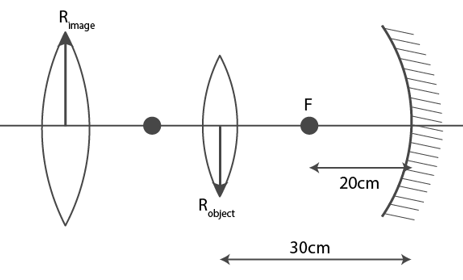Image of the circle formed at distance 60 cm in front of the mirror.

=> m = -v/u = [Radius of the image]/[Radius of the object]

=> -(-60)/(-30) = [Radius of the image]/2

=> Radius of the image = 4 cm

Hence, radius of the image formed = 4cm

Question 11: A concave mirror of radius R is kept on a horizontal table (figure below). Water (refractive index = μ) is poured into it up to a height h. Where should an object be placed so that its image is formed on itself?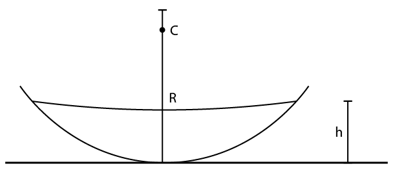Solution:

Let the object placed at a height x above the surface of water.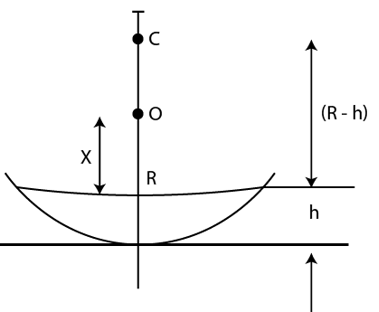If the image has to be formed at the same position, then the apparent position of the object with respect to mirror should be at the center of curvature.

=> [Real depth]/[apparent depth] = 1/μ

Now, x/(R-h) = 1/μ

=> x = [R-h]/μ

Question 12: A point source S is placed midway between two converging mirrors having equal focal length f as shown in figure (below). Find the values of d for which only one image is formed.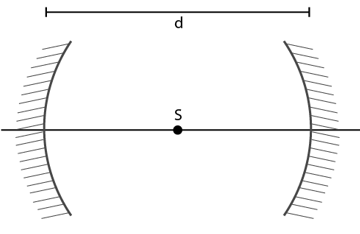Solution:

Both the mirrors have equal focal length f. (Given)

Case 1:

The source is at distance ‘2f’ from each mirror, that is the object is at center of curvature of the mirrors. Then the image will be produced at the same point S.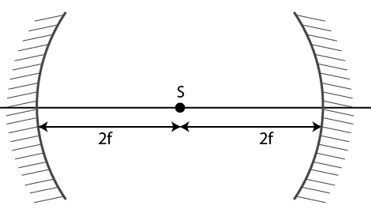=> d = 2f + 2f = 4f

Case 2:

When the source s is at a distance f from each mirror, the rays from the source after reflecting from one mirror will become parallel and so these parallel rays after the reflection from the other mirror the object itself. In this case only one image is formed.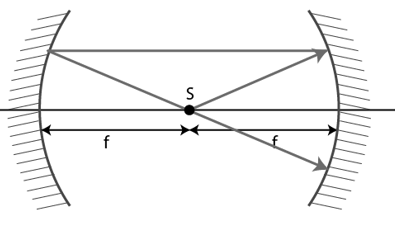=> d = f +f = 2f

Question 13: A converging mirror M1, a point source S and a diverging mirror M2 are arranged as shown in figure (below). The source is placed at a distance of 30 cm for M1. The focal length of each of the mirrors is 20 cm. Consider only the images formed by a maximum of two reflections. It is found that one image is formed on the source itself. (a) Find the distance between the two mirrors. (b) Find the location of the image formed by the single reflection from M2.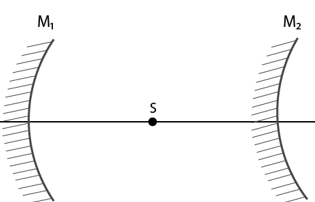Solution:

The focal length of both the mirrors is same, i.e. 20 cm, and the image is also formed at the focus after the reflection from both the mirror surfaces.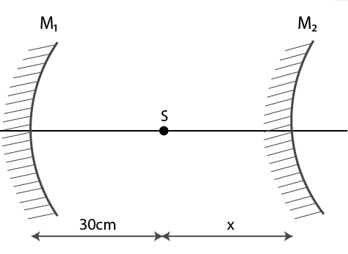For mirror M1:

The distance of object from the mirror, u= -30cm (converging mirror)

Focal length, f= -20cm

Using mirror formula,

1/v + 1/u = 1/f …(1)

Here u = 30 and f = -20

=> v = -60 cm

For mirror M2:

Object distance from the mirror will be the difference of the image formed by first mirror and the distance between the two mirrors.

=> u = 60 – (30 + x) cm = 30-x cm

Distance of image from the mirror, v= -x cm

Focal length of the mirror, f= 20cm

mirror formula => -1/x + 1/(30-x) = 1/20

=> x = 20 cm or -30 cm

Therefore, total distance between the two lines is 20+30 = 50 cm.

Question 14: A light ray falling at an angle of 45° with the surface of a clean slab of ice of thickness 1.00 m is refracted into it at an angle of 30°. Calculate the time taken by the light rays to cross the slab. Speed of light in vacuum = 3 x 108 ms-1.

Solution:

Angle of incidence i= 45°

Angle of refraction r= 30°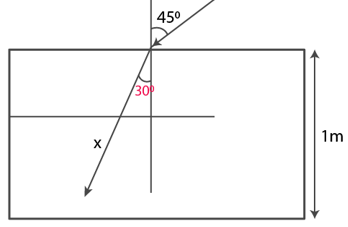Using the Snell’s law:

[sin i]/[sin r] = c/v

Where c = velocity of light in are and v = velocity of light in medium

=> [sin i]/[sin r] = [3×108]/v

=> sin 45o/sin30o = [3×108]/v

=> v = [3×108]/√2 m/s

Now, Distance travelled by light in the slab is x

x = 1/cos30o = 2/√3

Hence, time taken by light = 2√2/[√3 x 3 x 108]

= 5.4×10-9 sec

Question 15: A pole of length 1.00 m stands half dipped in a swimming pool with water level 50.0 cm higher than the bed. The refractive index of water is 1.33 and sunlight is coming at an angle of 45° with the vertical. Find the length of the shadow of the pole on the bed.

Solution:

Shadow length = BA’ = BD + A’D = 0.5 + 0.5 tan r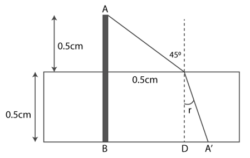Now, 1.33 = sin45o/sin r

=> sin r = 0.53

=> cos r = √(1-sin2r = √[1-(0.53)2] = 0.85

=> tan r = 0.6235

Therefore, shadow length = 0.5(1+0.6235) = 81.2 cm

Question 16: A small piece of wood is floating on the surface of a 2.5 m deep lake. Where does the shadow form on the bottom when the sun is just setting? Refractive index of water = 4/3.

Solution:

Height of the lake= 2.5m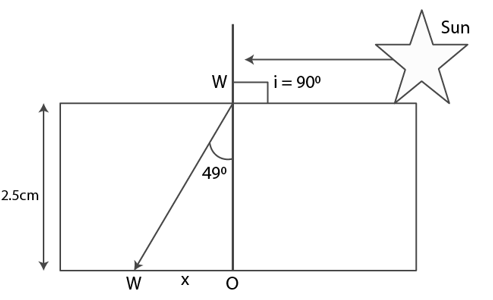Using the Snell’s law:

[sin i]/[sin r] = μ21 = (4/3)/1

=> sin r = 3/4

=> r = 49o

From diagram,

tan r = x/2.5

=> tan 49o = x/2.5

=> x = 2.8 m

Question 17: An object P is focused by a microscope M. A glass slab of thickness 2.1 cm is introduced between P and M. If the refractive index of the slab is 1.5, by what distance should the microscope be shifted to focus the object again?

Solution:

The thickness of the glass slab, d = 2 cm

and refractive index of the slab μ = 1.5

shift due to the glass slab: Δ T = (1−1/μ) d = (1−1/1.5)2.1 = 0.7 cm

Microscope should be shifted 0.70cm to focus the object again.

Question 18: A vessel contains water up to a height of 20 cm and above it an oil up to another 20 cm. The refractive indices of the water and the oil are 1.33 and 1.30 respectively. Find the apparent depth of the vessel when viewed from above.

Solution:

Let Δto is shift due to oil and Δtw = shift due to water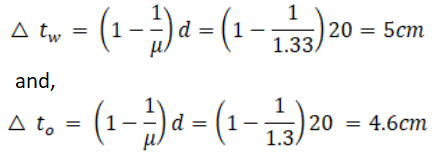Total shift Δt = 5 + 4.6 = 9.6 cm

Apparent depth = 40 – (9.6) = 30.4 cm below the surface.

Question 19: Locate the image of the point P as seen by the eye in the figure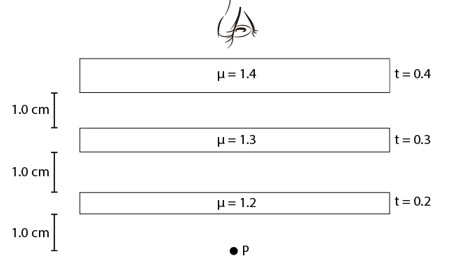Solution:

The presence of air medium in between the sheets does not affect the shift.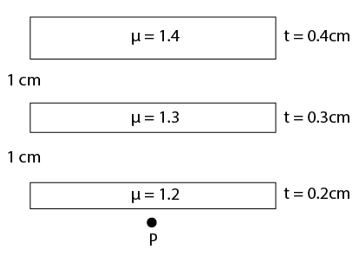Hence, the shift will be due to 3 sheets of different reactive index other than air

= (1 – 1/1.2)0.2 + (1- 1/13)(0.3) + (1- 1/14)(0.4)

= 0.2 cm above point P

Question 20: k transparent slabs are arranged one over another. The refractive indices of the slabs are μ1, μ2, μ3, … μk and the thickness are t1, t2, t3, … tk. An object is seen through this combination with nearly perpendicular light. Find the equivalent refractive index of the system which will allow the image to be formed at the same place.

Solution:

Total number of transparent slabs= k

Refractive index= μ1, μ2, μ3, … μk

Thickness of the slabs = t1, t2, t3, … tk

Let refractive index, μ, of combination of slabs and image is formed at same place.

Then the shift: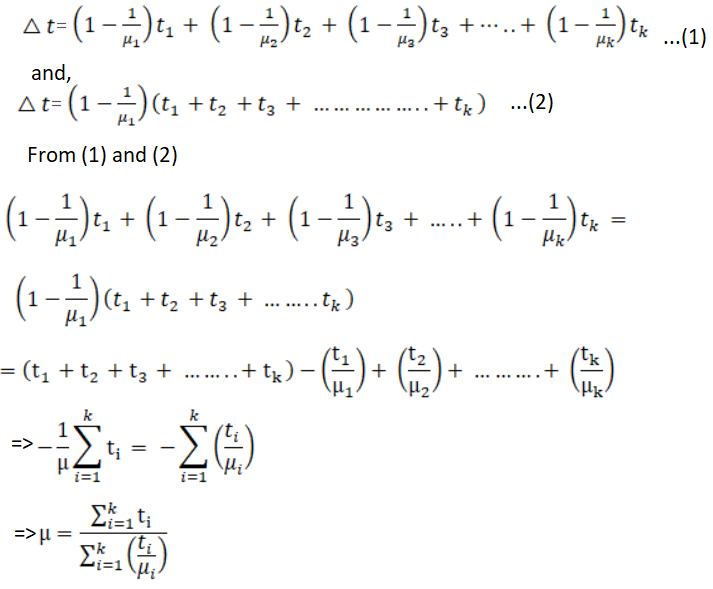Question 21: A cylindrical vessel of diameter 12 cm contains 800π cm3 of water. A cylindrical glass piece of diameter 8.0 cm and height 8.0 cm is placed in the vessel. If the bottom of the vessel under the glass piece is seen by the paraxial rays (see figure below), locate its image. The index of refraction of glass is 1.50 and that of water is 1.33.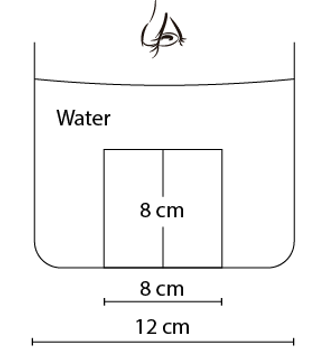Solution: Volume of water in cylindrical vessel = 800 π cm3

Volume of cylindrical water column after putting the glass piece inside it:

π r2h = 800π + π rg2 h1

rg = Radius of glass piece = 4 cm

h1 = Height of glass piece = 8cm

and r = 6 cm

=> h = 25.7 cm

There will two shifts due to the presence of glass and water:

1st shift for glass and 2nd shift for water are as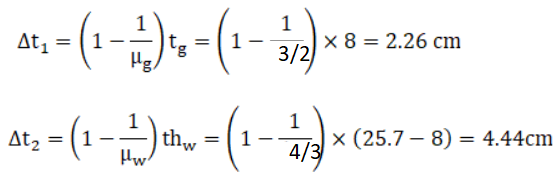Total shift = Δt_1 + Δt_2 = (2.26+ 4.44) cm= 7.1 cm above the bottom.

Question 22: Consider the situation in figure (below). The bottom of the pot is a reflecting plane mirror, S is a small fish and T is a human eye. Refractive index of water is μ.

(a) At what distance(s) from itself will the fish see the image(s) of the eye?

(b) At what distance(s) from itself will the eye see the image(s) of the fish?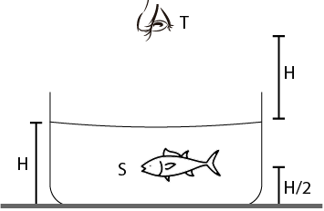Solution: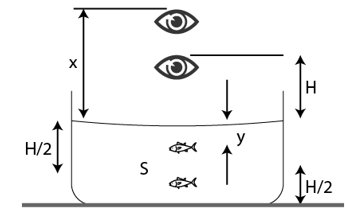(a)Let x be the distance of the image of the eye formed above the surface as seen by the fish

=> H/x = [Real depth]/[Apprent depth] = 1/μ

=> x = μH

and [H/2 + μH] is the distance of the direct image.

Similarly, [H/2 + (H+x)] is the distance of image through mirror

which implies [H/2 + (H+x)] = H(μ + 3/2)

(b) Here μ = (H/2)/y => y = H/2μ

Where y is the distance of the image of fish below the surface as seen by eye.

Direct image = H + y = H + H/2μ = H(1 + 1/2μ)

Another image of fish will be formed H/2 below the mirror. So, the real depth for that image of fish becomes 3H/2.

Apparent depth for the surface of water = 3H/2μ

Therefore, the distance of image from the eye is H + 3H/2μ = H(1 + 3/2μ)

Question 23: A small object is placed at the center of the bottom of a cylindrical vessel of radius 3 cm and height 4 cm filled completely with water. Consider the ray leaving the vessel through corner. Suppose this ray and the ray along the axis of the vessel is used to trace the image. Find the apparent depth and the ratio of real depth to apparent depth under the assumptions taken. Refractive index of water = 1.33.

Solution: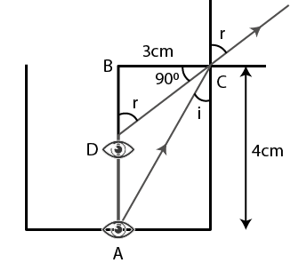By using Snell’s law;

sin i/sin r = 1/μw = 1/1.33 = 3/4

Where μw = Refractive Index of water = 1.33

=> sin r = (4/3) sin i …(A)

From the diagram, cos r = x/3 ….(1)

and sin i = 3/5

(A) => sin r = 4/5

Therefore, cot r = 3/4 …(2)

Equating (1) and (2)

x/3 = 3/4

=> x = 9/4 = 2.25 cm; apparent depth

Hence, The ratio of real depth to apparent depth = 4 : (2.25) = 1.78.

Question 24: A cylindrical vessel, whose diameter and height both are equal to 30 cm, is placed on a horizontal surface and a small particle P is placed in it at a distance of 5.0 cm from the center. An eye is placed at a position such that the edge of the bottom is just visible ( figure below). The particle P is in the plane of drawing. Up to what minimum height should water be poured in the vessel to make the particle P visible?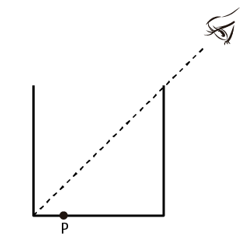Solution: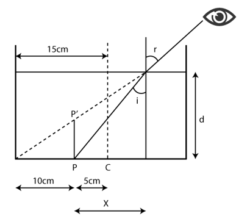For the given cylindrical vessel;

Diameter (d) = 30cm, Radius (r) = 15 cm, Height (h) = 30 cm and d = height of water

By using Snell’s law;

sin i/sin r = 1/μw = 1/1.33 = 3/4

Where μw = Refractive Index of water = 1.33

here Angle of refraction = 45^o

=> sin i = 3/(4√2)

The Point “P” will be visible when the refracted ray makes angle of 45 degree at the point of refraction.

Let x cm distance from P to X.

tan 45o = (x+10)/d

=> d = x + 10 …(1)

Now, tan i = x/d

Using value of sin i, we have the value of tan i = 3/√23

=> 3/√23 = (d-10)/d

=> d = 26.7 cm

Question 25: A light ray is incident at an angle of 45° with the normal to a√2 cm thick plate (μ = 2.0). Find the shift in the path of the light as it emerges out from the plate.

Solution: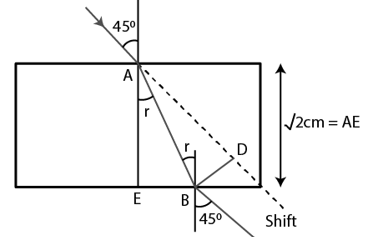From the diagram,

(sin 45o)/sin r = 2/1

=> sin r = 1/(2√2)

or r = 21o

Here θ = (45o – 21 o) = 24 o

and BD = shift in path = AB sin 24 o

= 0.406 x AB

= AE/cos21 o x 0.406

= 0.62 cm

Question 26: An optical fibre (μ = 1.72) is surrounded by a glass coating (μ = 1.50). Find the critical angle for total internal reflection at the fibre-glass interface.

Solution:

By Snell’s law;

sin i/sin r = sinθc/sin90 o = μgo

Where μo = Refractive index of optical fibre = 1.72

and μg = Refractive index of glass coating = 1.50

Also, θc = critical angle = sin-1(1/μ)

=> sin θc/sin 90o = 1.50/1.72 = 75/86

=> θc = 75/86

Therefore, critical angle is sin-1(75/86)

Question 27: A light ray is incident normally on the face AB of a right-angled prism ABC (μ = 1.50) as shown in figure (below). What is the largest angle ϕ for which the light ray is totally reflected at the surface AC?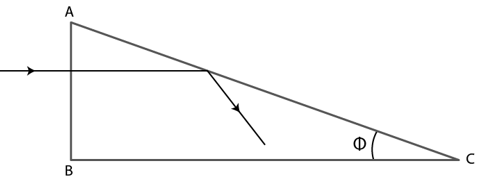Solution: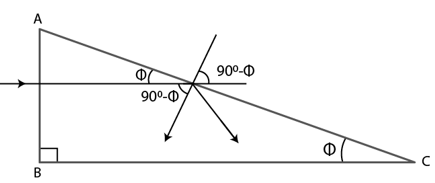By Snell’s law;

sin i/sin r = 1/μ

Also, θc = critical angle = sin-1(1/μ)

=> sin θc/sin 90o = 1/μ = 1/1.50 = 2/3

=> θc = sin-1(2/3)

Now, for total internal reflection, 90o – φ > θc (from diagram)

=> φ < 90o – θc = cos-1(2/3)

Thus, largest angle φ for which the light ray is totally reflected at the surface,

AC = cos-1(2/3)

Question 28: Find the maximum angle of refraction when a light ray is refracted from glass (μ = 1.50) to air.

Solution:

From the definition of critical angle, if a refracted ray makes an angle more than 90 degrees, then total internal reflection will occur. So, the maximum angle of refraction is 90 degrees.

Question 29: Light is incident from glass (μ = 1.5) to air. Sketch the variation of the angle of deviation δ with the angle of incident i for 0 < I < 90°.

Solution: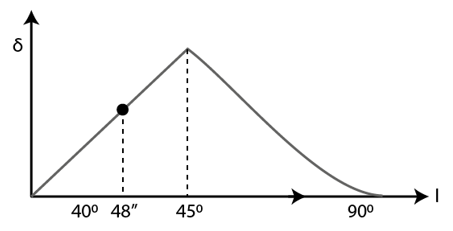Θc = critical angle = sin-1(1/μ)

and sin θc/sin r = μag

Here μa = 1.0 and μg = 1.5 and r = 90o

=> sin θc/sin90o = 2/3

=> θc = 40o48”

The angle of deviation due to refraction from glass to air increases as the angle of incidence increases from 0° to 40°48”. The angle of deviation due to total internal reflection further increases for 40°48” to 45° and then it decreases.

Question 30: Light is incident from glass (μ = 1.50) to water (μ = 1.33). Find the range of the angle of deviation for which there are two angles of incidence.

Solution: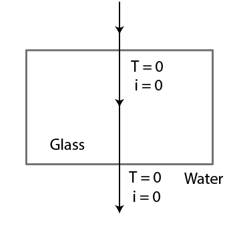For two angles of incidence,

1) When light passes through the normal:

Angle of incidence = 0o, Angle of refraction= 0 o and Angle of deviation = 0 o

(2)When light incident at critical angle :

sin θc/sin r = μwg

here μW = 1.33 = 4/3 and μg = 1.5 = 3/2

Therefore, sin θc = 8/9

and θc = sin-1(8/9)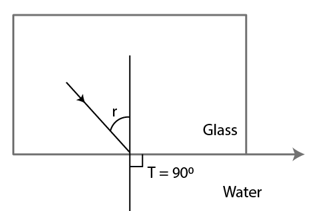Also, the Angle of deviation = 90 o – θc

= 90 o – sin-1 (8/9)

= cos-1 (8/9)

So, If angle of incidence increases beyond critical angle, total internal reflection will occur.

Thus, the range of angle of deviation is from 0 o to cos-1(8/9).

Question 31: Light falls from glass (μ = 1.5) to air. Find the angle of incidence for which the angle of deviation is 90°.

Solution:

sin θc = 8/9

We know, critical angle = θc = sin-1(1/μ) = 41.8o

Here μ = 1.5

Maximum angle of deviation in refraction = 90o – 41.8o = 47.2 o

In this case, total internal reflection must have taken place. In reflectio, deviation = 180 o – 2i = 90 o

=> i = 45 o

Question 32: A point source is placed at a depth h below the surface of water (refractive index = μ).

(a) Show that light escapes through a circular area on the water surface with its centre directly above the point source.

(b) Find the angle subtended by a radius of the area on the source.

Solution:

(a)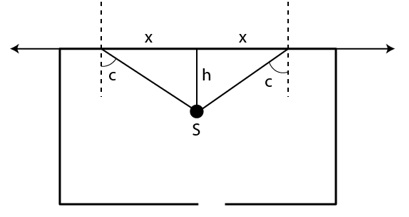Here S be the source point and x be the radius of circular area.

We know, critical angle = θc

So, tan θc = x/h

=>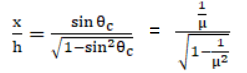[we know, sin θc = (1/μ)]

=> x = h/√(μ2-1)

Light escapes from the circular area at a fixed desistance r on the water surface, directly above the point source S.

(b) As, sin θc = (1/μ)

=> θc = sin-1(1/μ)

Question 33: A container contains water up to a height of 20 cm and there is a point source at the center of the bottom of the container. A rubber ring of radius r floats centrally on the water. The ceiling of the room is 2.0 m above the water surface.

(a) Find the radius of the shadow of the ring formed on the ceiling if r = 15 cm.

(b) Find the maximum value of r for which the shadow of the ring is formed on the ceiling. Refractive index of water = 4/3.

Solution: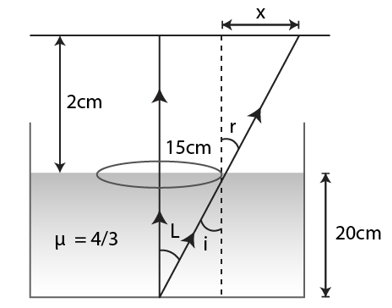(a)Height of water container= h= 20 cm ..(given)

Ceiling of the room is 2.0 m above the water surface. ..(given)

From diagram, sin i = 15/25

By Snell’s law,

sin i/sin r = 1/μ = 3/4

[Refractive index of water = 4/3 (given]

=> sin i = 4/5

Again tan r = x/2 (from diagram)

Therefore,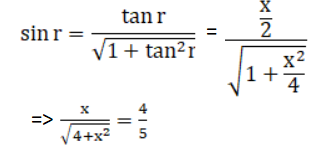=> x = 8/3 cm

Total radius of the shadow = 8/3 + 0.15 = 2.81 m

(b) For Maximum angle of refraction: i = θc

Let R is the maximum radius.

sin θc = sin i/sin r

=> sin θc = sin θc /sin r

=> R/ √[R^2+20] = ¾

=>16R2 = 9R2 + 9×400

=>R = 22.67 cm

Question 34: Find the angle of minimum deviation for an equilateral prism made of a material of refractive index 1.732. What is the angle of incidence for this deviation?

Solution: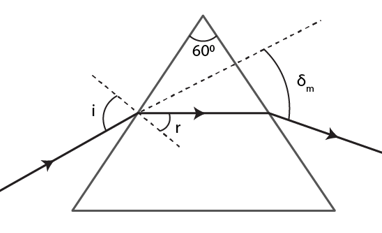Let Angle of prism = A and δm is the minimum deviation.

=> 1.732 x sin 30 o = sin[(Angle of minimum deviation +60 o)/2]

=> 1.732 x (1/2) = sin[δm +60 o)/2]

[Here 1.732 x sin 30o = √3/2 which is equal to sin 60 o]

So, sin 60o = sin[(δm+60 o)/2]

or δm + 60 o = 2 x 60 o

=> δm = 60 o

Also, δm = 2i – A

=> 2i = 120 o

or i = 60 o

Therefore, the minimum angle of deviation, δm = 60 o.

Required angle of incidence = i = 60 o

Question 35: Find the angle of deviation suffered by the light ray shown in figure (below). The refractive index μ = 1.5 for the prism material.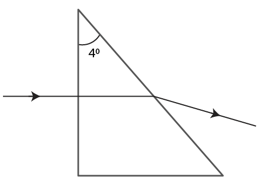Solution: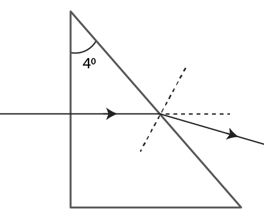We know, Angle of minimum deviation,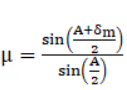Find angle of deviation, δm

For small angle, sin θ = θ (approx.)]

=>μ = [(A+δm)/2]/[sin(A/2)]

Here A = 40

=> 1.5 = [40 + δm]/2o

Given μ = 1.5

=> δm = 2o